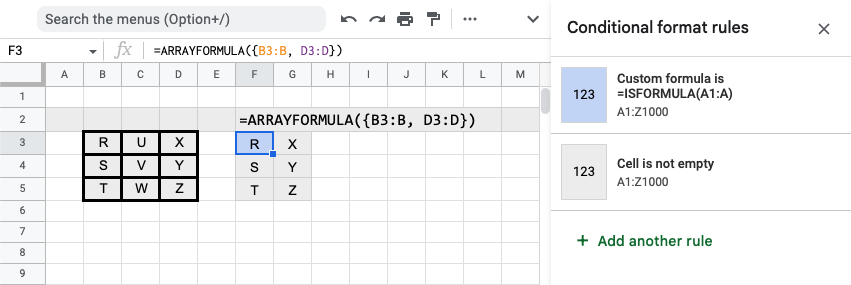Insert a range of rows from another sheet in Google Sheets.

Use `ARRAYFORMULA()` instead of `IMPORTRANGE()` when referencing the a sheet in the same spreadsheet as `ARRAYFORMULA()` is faster.

Here's how to pass an array (using brackets) to reference multiple ranges:

``=ARRAYFORMULA({MySheet!B3:B, MySheet!D3:D})``

If you just need to reference a basic range, use something like this:

``=ARRAYFORMULA(MySheet!B3:B)``

Slower using `IMPORTRANGE()`, but needed if referencing a sheet from a different spreadsheet:

``=IMPORTRANGE("https://docs.google.com/spreadsheets/d/01234567890/", "MySheet!B3:B")``